### Home > CALC > Chapter 6 > Lesson 6.2.2 > Problem6-74

6-74.
1. From her second floor window 6 meters above the ground, Xiomara throws a tennis ball at a rate of 11 meters per second up towards her friend Itzagueri who is 12 meters above the ground. Assume a(t) = −9.8 meters per sec2 for the acceleration due to gravity. Homework Help ✎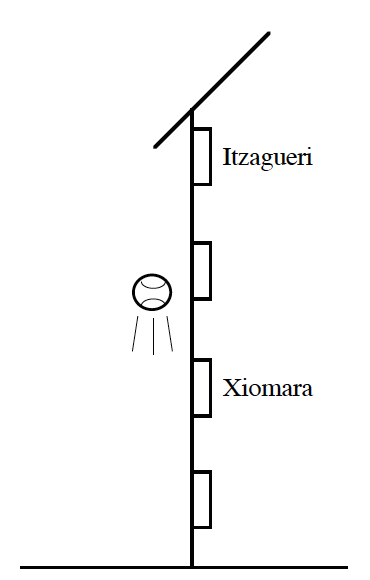1. Assuming Itzagueri does not catch the ball, describe the motion of the ball 2 seconds after it was thrown.

2. When does the ball reach its highest point?

4. Determine how high the ball is when it is falling at the rate of 5 meters per second.

5. Explain what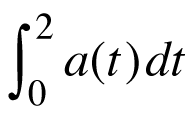represents physically.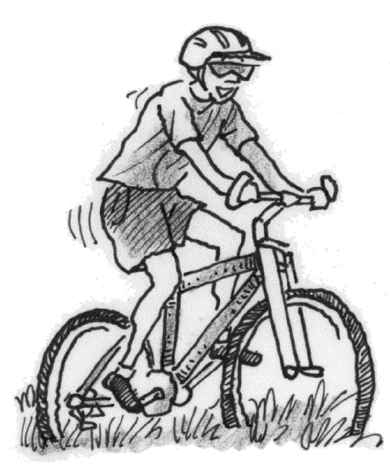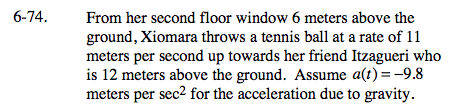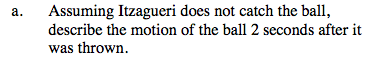At t = 2, is the ball rising or falling? Is it's velocity increasing or decreasing?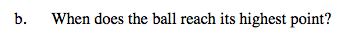The ball stops moving at its highest point.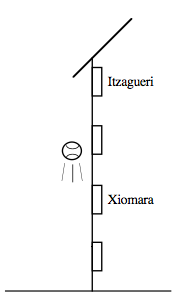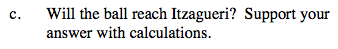The height is given by h(t) = –4.9t2 + 11t + 6.
h(answer from part (b)) ≈ 12.173 m; so yes.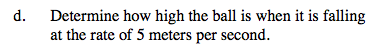Before you evaluate the height, find the time when
v(t) = −5.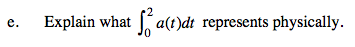Be sure to explain the significance of BOTH the integrand and the bounds.